# Working with and Writing Data

The following sections explain how to write various types of data to an Excel worksheet using XlsxWriter.

## Writing data to a worksheet cell

The `worksheet` `write()` method is the most common means of writing Python data to cells based on its type:

```import xlsxwriter

workbook = xlsxwriter.Workbook('write_data.xlsx')

worksheet.write(0, 0, 1234)     # Writes an int
worksheet.write(1, 0, 1234.56)  # Writes a float
worksheet.write(2, 0, 'Hello')  # Writes a string
worksheet.write(3, 0, None)     # Writes None
worksheet.write(4, 0, True)     # Writes a bool

workbook.close()
```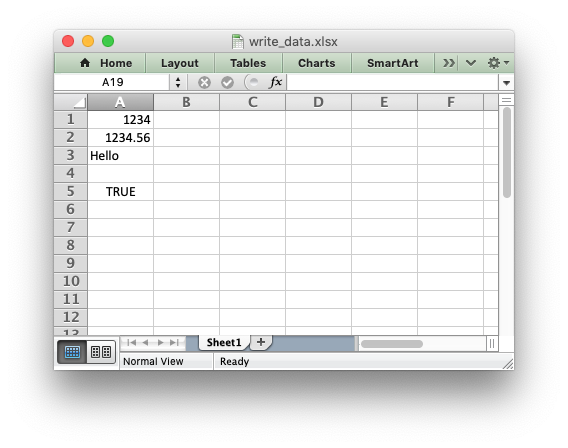The `write()` method uses the `type()` of the data to determine which specific method to use for writing the data. These methods then map some basic Python types to corresponding Excel types. The mapping is as follows:

Python type Excel type Worksheet methods
`int` Number `write()`, `write_number()`
`long`
`float`
`Decimal`
`Fraction`
`basestring` String `write()`, `write_string()`
`str`
`unicode`
`None` String (blank) `write()`, `write_blank()`
`datetime.date` Number `write()`, `write_datetime()`
`datetime.datetime`
`datetime.time`
`datetime.timedelta`
`bool` Boolean `write()`, `write_boolean()`

The `write()` method also handles a few other Excel types that are encoded as Python strings in XlsxWriter:

Pseudo-type Excel type Worksheet methods
formula string Formula `write()`, `write_formula()`
url string URL `write()`, `write_url()`

It should be noted that Excel has a very limited set of types to map to. The Python types that the `write()` method can handle can be extended as explained in the Writing user defined types section below.

## Writing unicode data

Unicode data in Excel is encoded as UTF-8. XlsxWriter also supports writing UTF-8 data. This generally requires that your source file is UTF-8 encoded:

```worksheet.write('A1', 'Some UTF-8 text')
```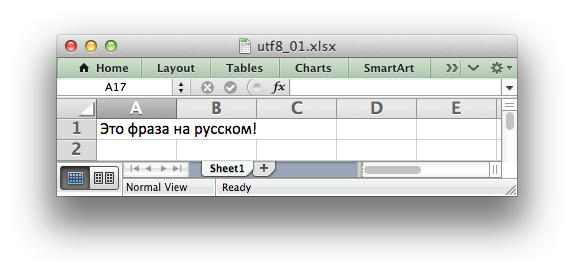See Example: Simple Unicode with Python 3 for a more complete example.

Alternatively, you can read data from an encoded file, convert it to UTF-8 during reading and then write the data to an Excel file. See Example: Unicode - Polish in UTF-8 and Example: Unicode - Shift JIS.

## Writing lists of data

Writing compound data types such as lists with XlsxWriter is done the same way it would be in any other Python program: with a loop. The Python `enumerate()` function is also very useful in this context:

```import xlsxwriter

workbook = xlsxwriter.Workbook('write_list.xlsx')

my_list = [1, 2, 3, 4, 5]

for row_num, data in enumerate(my_list):
worksheet.write(row_num, 0, data)

workbook.close()
```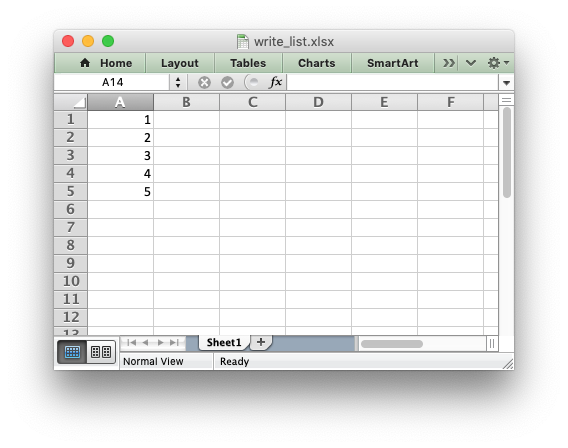Or if you wanted to write this horizontally as a row:

```import xlsxwriter

workbook = xlsxwriter.Workbook('write_list.xlsx')

my_list = [1, 2, 3, 4, 5]

for col_num, data in enumerate(my_list):
worksheet.write(0, col_num, data)

workbook.close()
```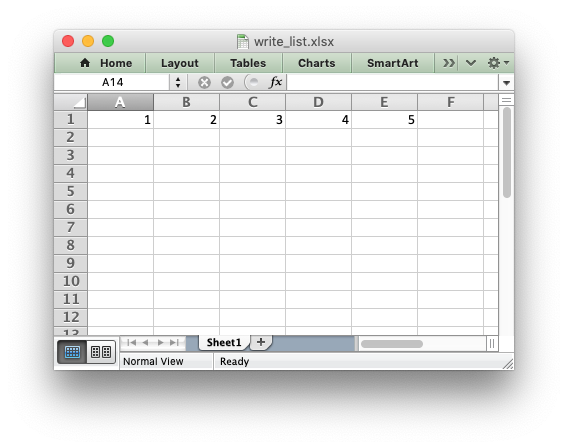For a list of lists structure you would use two loop levels:

```import xlsxwriter

workbook = xlsxwriter.Workbook('write_list.xlsx')

my_list = [[1, 1, 1, 1, 1],
[2, 2, 2, 2, 1],
[3, 3, 3, 3, 1],
[4, 4, 4, 4, 1],
[5, 5, 5, 5, 1]]

for row_num, row_data in enumerate(my_list):
for col_num, col_data in enumerate(row_data):
worksheet.write(row_num, col_num, col_data)

workbook.close()
```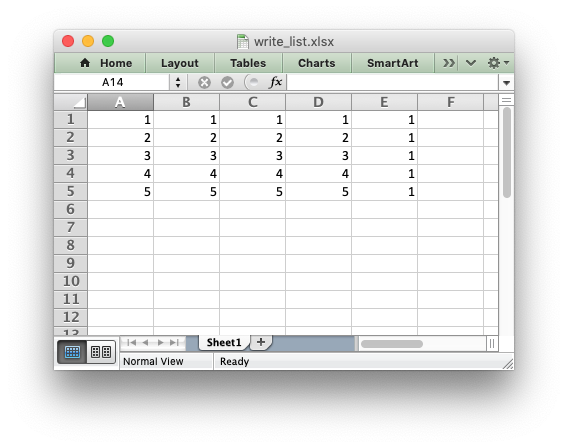The worksheet class has two utility functions called `write_row()` and `write_column()` which are basically a loop around the `write()` method:

```import xlsxwriter

workbook = xlsxwriter.Workbook('write_list.xlsx')

my_list = [1, 2, 3, 4, 5]

worksheet.write_row(0, 1, my_list)
worksheet.write_column(1, 0, my_list)

workbook.close()
```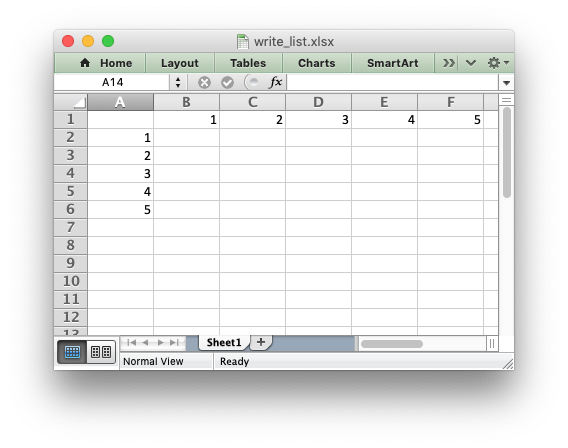## Writing dicts of data

Unlike lists there is no single simple way to write a Python dictionary to an Excel worksheet using Xlsxwriter. The method will depend of the structure of the data in the dictionary. Here is a simple example for a simple data structure:

```import xlsxwriter

workbook = xlsxwriter.Workbook('write_dict.xlsx')

my_dict = {'Bob': [10, 11, 12],
'Ann': [20, 21, 22],
'May': [30, 31, 32]}

col_num = 0
for key, value in my_dict.items():
worksheet.write(0, col_num, key)
worksheet.write_column(1, col_num, value)
col_num += 1

workbook.close()
```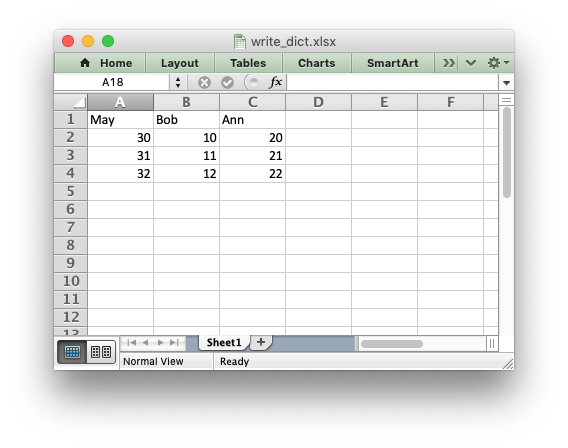## Writing dataframes

The best way to deal with dataframes or complex data structure is to use Python Pandas. Pandas is a Python data analysis library. It can read, filter and re-arrange small and large data sets and output them in a range of formats including Excel.

To use XlsxWriter with Pandas you specify it as the Excel writer engine:

```import pandas as pd

# Create a Pandas dataframe from the data.
df = pd.DataFrame({'Data': [10, 20, 30, 20, 15, 30, 45]})

# Create a Pandas Excel writer using XlsxWriter as the engine.
writer = pd.ExcelWriter('pandas_simple.xlsx', engine='xlsxwriter')

# Convert the dataframe to an XlsxWriter Excel object.
df.to_excel(writer, sheet_name='Sheet1')

# Close the Pandas Excel writer and output the Excel file.
writer.close()
```

The output from this would look like the following: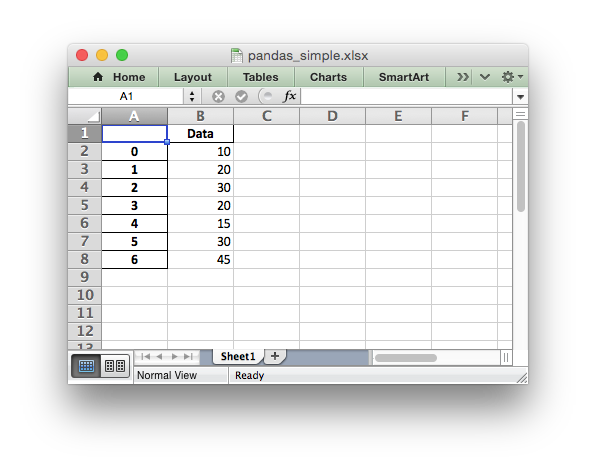For more information on using Pandas with XlsxWriter see Working with Pandas and XlsxWriter.

## Writing user defined types

As shown in the first section above, the worksheet `write()` method maps the main Python data types to Excel’s data types. If you want to write an unsupported type then you can either avoid `write()` and map the user type in your code to one of the more specific write methods or you can extend it using the `add_write_handler()` method. This can be, occasionally, more convenient then adding a lot of if/else logic to your code.

As an example, say you wanted to modify `write()` to automatically write `uuid` types as strings. You would start by creating a function that takes the uuid, converts it to a string and then writes it using `write_string()`:

```def write_uuid(worksheet, row, col, uuid, format=None):
return worksheet.write_string(row, col, str(uuid), format)
```

You could then add a handler that matches the `uuid` type and calls your user defined function:

```#                           match,     action()
```

Then you can use `write()` without further modification:

```my_uuid = uuid.uuid3(uuid.NAMESPACE_DNS, 'python.org')

# Write the UUID. This would raise a TypeError without the handler.
worksheet.write('A1', my_uuid)
```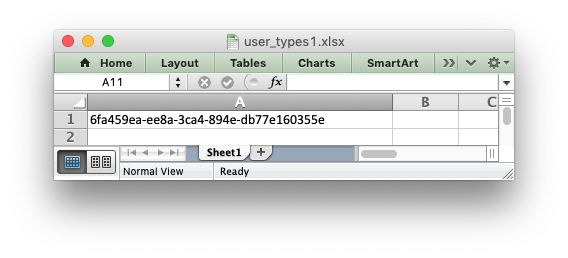Multiple callback functions can be added using `add_write_handler()` but only one callback action is allowed per type. However, it is valid to use the same callback function for different types:

```worksheet.add_write_handler(int,   test_number_range)
```

### How the write handler feature works

The `write()` method is mainly a large `if()` statement that checks the `type()` of the input value and calls the appropriate worksheet method to write the data. The `add_write_handler()` method works by injecting additional type checks and associated actions into this `if()` statement.

Here is a simplified version of the `write()` method:

```def write(self, row, col, *args):

# The first arg should be the token for all write calls.
token = args

# Get the token type.
token_type = type(token)

# Check for any user defined type handlers with callback functions.
if token_type in self.write_handlers:
write_handler = self.write_handlers[token_type]
function_return = write_handler(self, row, col, *args)

# If the return value is None then the callback has returned
# control to this function and we should continue as
# normal. Otherwise we return the value to the caller and exit.
if function_return is None:
pass
else:
return function_return

# Check for standard Python types, if we haven't returned already.
if token_type is bool:
return self.write_boolean(row, col, *args)

# Etc. ...
```

### The syntax of write handler functions

Functions used in the `add_write_handler()` method should have the following method signature/parameters:

```def my_function(worksheet, row, col, token, format=None):
return worksheet.write_string(row, col, token, format)
```

The function will be passed a worksheet instance, an integer `row` and `col` value, a `token` that matches the type added to `add_write_handler()` and some additional parameters. Usually the additional parameter(s) will only be a cell format instance. However, if you need to handle other additional parameters, such as those passed to `write_url()` then you can have more generic handling like this:

```def my_function(worksheet, row, col, token, *args):
return worksheet.write_string(row, col, token, *args)
```

Note, you don’t have to explicitly handle `A1` style cell ranges. These will be converted to row and column values prior to your function being called.

You can also make use of the `row` and `col` parameters to control the logic of the function. Say for example you wanted to hide/replace user passwords with ‘****’ when writing string data. If your data was structured so that the password data was in the second column, apart from the header row, you could write a handler function like this:

```def hide_password(worksheet, row, col, string, format=None):
if col == 1 and row > 0:
return worksheet.write_string(row, col, '****', format)
else:
return worksheet.write_string(row, col, string, format)
```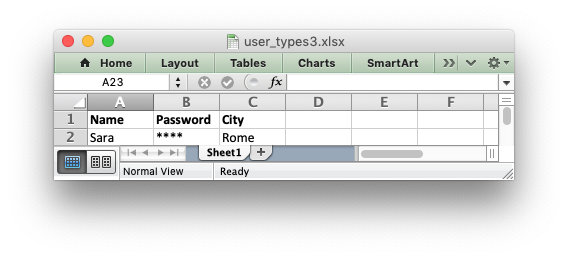### The return value of write handler functions

Functions used in the `add_write_handler()` method should return one of the following values:

• `None`: to indicate that control is return to the parent `write()` method to continue as normal. This is used if your handler function logic decides that you don’t need to handle the matched token.
• The return value of the called `write_xxx()` function. This is generally 0 for no error and a negative number for errors. This causes an immediate return from the calling `write()` method with the return value that was passed back.

For example, say you wanted to ignore `NaN` values in your data since Excel doesn’t support them. You could create a handler function like the following that matched against floats and which wrote a blank cell if it was a `NaN` or else just returned to `write()` to continue as normal:

```def ignore_nan(worksheet, row, col, number, format=None):
if math.isnan(number):
return worksheet.write_blank(row, col, None, format)
else:
# Return control to the calling write() method.
return None
```

If you wanted to just drop the `NaN` values completely and not add any formatting to the cell you could just return 0, for no error:

```def ignore_nan(worksheet, row, col, number, format=None):
if math.isnan(number):
return 0
else:
# Return control to the calling write() method.
return None
```

### Write handler examples

See the following, more complete, examples of handling user data types: